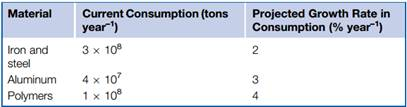### Create an Account

Already have account?

### Forgot Your Password ?

Home / Questions / Commodity A is currently consumed at the rate CA tons per year and commodity B at the rate...

# Commodity A is currently consumed at the rate CA tons per year and commodity B at the rate CB tons per year CA CB If the two consumption rates are increasing exponentially to give growths in

Commodity A is currently consumed at the rate CA tons per year, and commodity B at the rate CB tons per year (CA >CB). If the two consumption rates are increasing exponentially to give growths in consumption after each year of rA% and rB%, respectively (rA <>B), derive an equation for the time, measured from the present day, before the annual consumption of B exceeds that of A.

b. The table shows figures for consumption and growth rates of steel, aluminum, and plastics. What are the doubling-times (in years) for consumption of these commodities?

c. Calculate the number of years before the consumption of (a) aluminum and (b) polymers would exceed that of steel, if exponential growth continued.Jun 26 2020 View more View Less

#### Answer (Solved)Subscribe To Get Solution